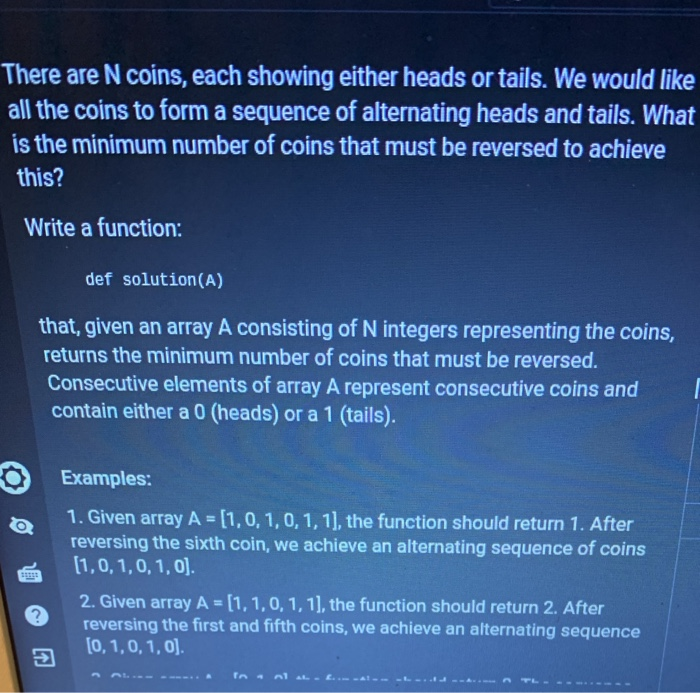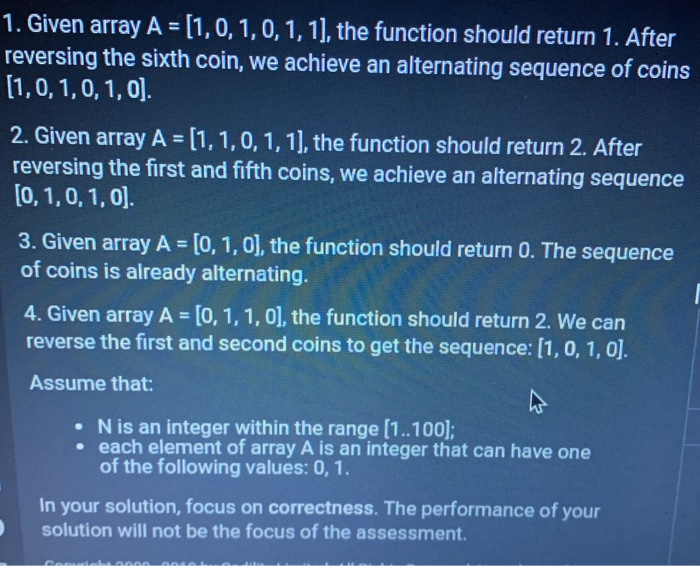# (Solved) : N Coins Showing Either Heads Tails Would Like Coins Form Sequence Alternating Heads Tails Q41065762 . . .

\$9.00There are N coins, each showing either heads or tails. We would like all the coins to form a sequence of alternating heads and tails. What is the minimum number of coins that must be reversed to achieve this? Write a function: def solution(A) that, given an array A consisting of N integers representing the coins, returns the minimum number of coins that must be reversed. Consecutive elements of array A represent consecutive coins and contain either a 0 (heads) or a 1 (tails). Examples: 1. Given array A = (1,0,1,0,1,1), the function should return 1. After reversing the sixth coin, we achieve an alternating sequence of coins (1,0,1,0, 1, 0). 2. Given array A = [1,1,0, 1, 1), the function should return 2. After reversing the first and fifth coins, we achieve an alternating sequence [0,1,0,1,0) 1. Given array A = [1,0, 1, 0, 1, 1), the function should return 1. After reversing the sixth coin, we achieve an alternating sequence of coins (1,0,1,0,1,0). 2. Given array A = [1,1,0,1,1). the function should return 2. After reversing the first and fifth coins, we achieve an alternating sequence [0,1,0, 1, 0]. 3. Given array A = [0, 1, 0], the function should return O. The sequence of coins is already alternating. 4. Given array A = [0, 1, 1, 0], the function should return 2. We can reverse the first and second coins to get the sequence: (1,0, 1,0). Assume that: N is an integer within the range (1..100); • each element of array A is an integer that can have one of the following values:0, 1. In your solution, focus on correctness. The performance of your solution will not be the focus of the assessment. Show transcribed image text There are N coins, each showing either heads or tails. We would like all the coins to form a sequence of alternating heads and tails. What is the minimum number of coins that must be reversed to achieve this? Write a function: def solution(A) that, given an array A consisting of N integers representing the coins, returns the minimum number of coins that must be reversed. Consecutive elements of array A represent consecutive coins and contain either a 0 (heads) or a 1 (tails). Examples: 1. Given array A = (1,0,1,0,1,1), the function should return 1. After reversing the sixth coin, we achieve an alternating sequence of coins (1,0,1,0, 1, 0). 2. Given array A = [1,1,0, 1, 1), the function should return 2. After reversing the first and fifth coins, we achieve an alternating sequence [0,1,0,1,0)
1. Given array A = [1,0, 1, 0, 1, 1), the function should return 1. After reversing the sixth coin, we achieve an alternating sequence of coins (1,0,1,0,1,0). 2. Given array A = [1,1,0,1,1). the function should return 2. After reversing the first and fifth coins, we achieve an alternating sequence [0,1,0, 1, 0]. 3. Given array A = [0, 1, 0], the function should return O. The sequence of coins is already alternating. 4. Given array A = [0, 1, 1, 0], the function should return 2. We can reverse the first and second coins to get the sequence: (1,0, 1,0). Assume that: N is an integer within the range (1..100); • each element of array A is an integer that can have one of the following values:0, 1. In your solution, focus on correctness. The performance of your solution will not be the focus of the assessment.There ... View full description